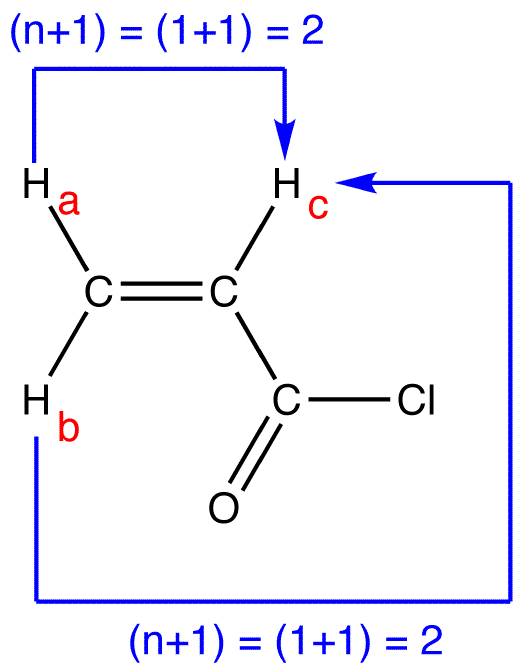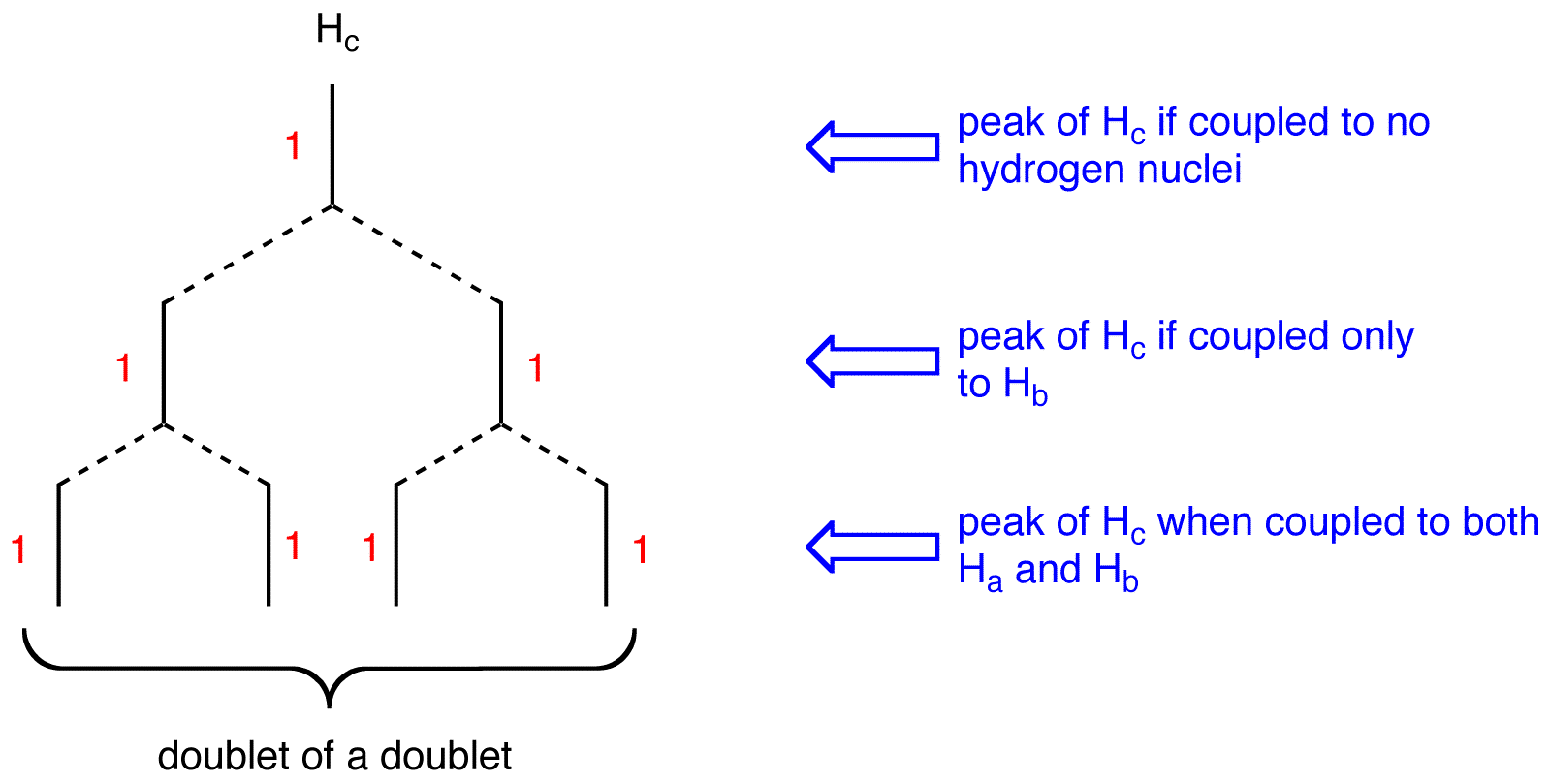# (n+1) Rule

•• Contributed by Gamini Gunawardena
• Associate Professor (Chemistry) at Utah Valley University

The (n+1) Rule, an empirical rule used to predict the multiplicity and, in conjunction with Pascal’s triangle, splitting pattern of peaks in 1H and 13C NMR spectra, states that if a given nucleus is coupled (see spin coupling) to n number of nuclei that are equivalent (see equivalent ligands), the multiplicity of the peak is n+1.

eg. 1:The three hydrogen nuclei in 1, Ha, Hb, and Hc, are equivalent. Thus, 1H NMR spectrum of 1 has only one peak. Ha, Hb, and Hc are coupled to no hydrogen nuclei. Thus, for Ha, Hb, and Hc, n=0; (n+1) = (0+1) = 1. The multiplicity of the peak of Ha, Hb, and Hc is one. The peak has one line; it is a singlet.

eg. 2:There are two sets of equivalent hydrogen nuclei in 2:

Set 1: Ha
Set 2: Hb, Hc

Thus, the 1H NMR spectrum of 2 has two peaks, one due to Ha and the other to Hb and Hc.

The peak of Ha: There are two vicinal hydrogens to Ha: Hb and Hc. Hb and Hc are equivalent to each other but not to Ha. Thus, for Ha, n=2; (n+1) = (2+1) = 3. The multiplicity of the peak of Ha is three. The peak has three lines; from the Pascal’s triangle, it is a triplet.

The peak of Hb and Hc: There is only one vicinal hydrogen to Hb and Hc: Ha. Ha is not equivalent to Hb and Hc. Thus, for Hb and Hc, n=1; (n+1) = (1+1) = 2. The multiplicity of the peak of Hb and Hc is two. The peak has two lines, from the Pascal’s triangle, it is a doublet.

To determine the multiplicity of a peak of a nucleus coupled to more than one set of equivalent nuclei, apply the (n+1) Rule independently to each other.

eg:There are three set of equivalent hydrogen nuclei in 3:

Set 1: Ha
Set 2: Hb
Set 3: Hcpeak of Ha:multiplicity of the peak of Ha = 2×2 = 4. To determine the splitting pattern of the peak of Ha, use the Pascal’s triangle, based on the observation that, for alkenyl hydrogens, Jcis > Jgem.The peak of Ha is a doublet of a doublet.

peak of Hb:multiplicity of the peak of Hb = 2×2 = 4. To determine the splitting pattern of the peak of Hb, use the Pascal’s triangle, based on the observation that, for alkenyl hydrogens, Jtrans > Jgem.The peak of Hb is a doublet of a doublet.

peak of Hc:multiplicity of the peak of Hc = 2×2 = 4. To determine the splitting pattern of the peak of Hc, use the Pascal’s triangle based on the observation that, for alkenyl hydrogens, Jtrans > Jcis.The peak of Hc is a doublet of a doublet.